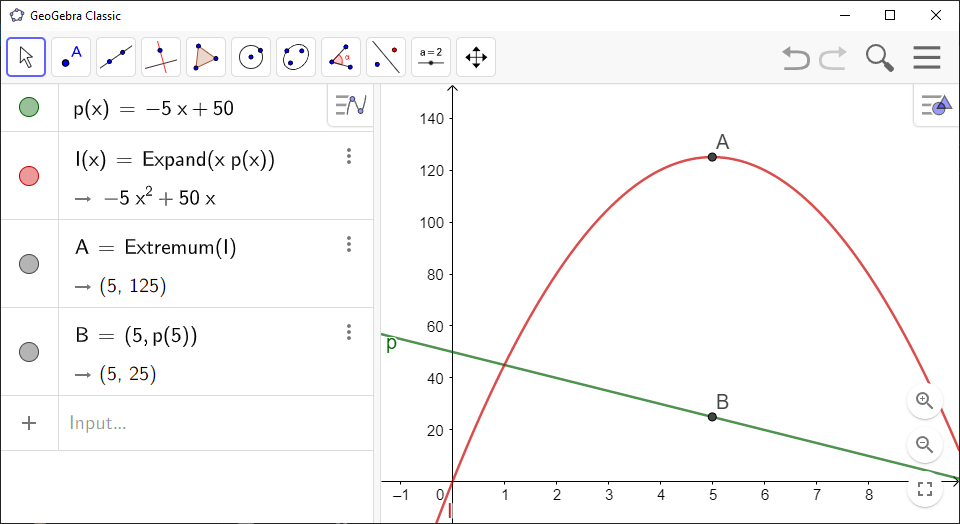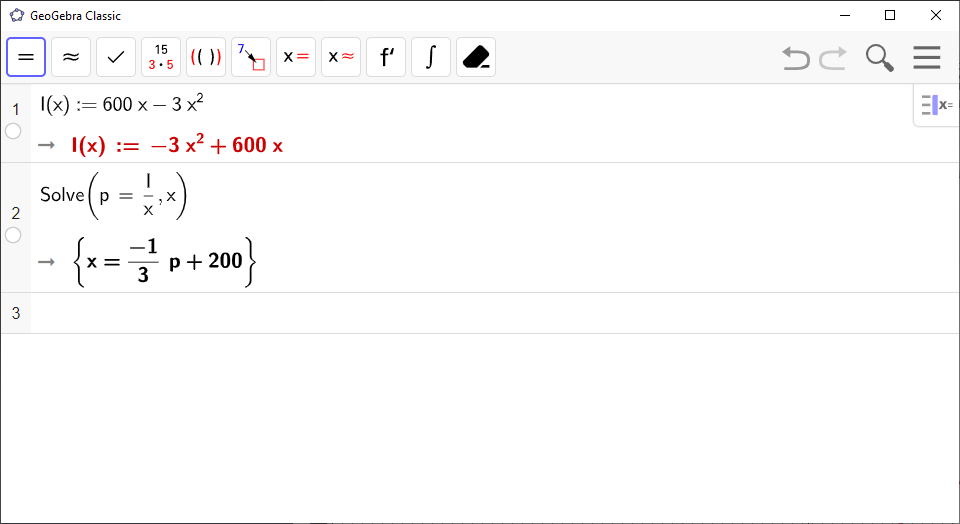# Revenue with Demand and Price in GeoGebra

You can use `GeoGebra` to find the revenue function and the demand.

• If you want to find the revenue function $R\left(x\right)$ when you have the price function $p\left(x\right)$, go to Item 3.

• If you want to find the demand that yields the greatest profit, and also use it to find the greatest revenue and the price of the product, go to Item 3.

• If you want to find the demand when you have the revenue function $R\left(x\right)$, go to Item 3.

`GeoGebra` Instruction 1

### Find$R\left(x\right)$WhenYouHave$p\left(x\right)$

1.
Open `Algebra View` under`View` in`Menu`.
2.
Enter the price function $p\left(x\right)$ in an empty row in `Algebra View`, like this:

p(x) = <Expression>

where you replace `<Expression>` with your expression. Press `Enter`.

3.
In a new row in `Algebra View`, type

R(x) = Expand(x * p(x))

Press `Enter`. Your revenue function $R\left(x\right)$ is displayed in the same row.

`GeoGebra` Instruction 2

### FindtheDemandthatYieldstheGreatestProfit

1.
Use Item 3 to get both the revenue function $R\left(x\right)$ and the price function $p\left(x\right)$ in `GeoGebra`.
2.
Find the greatest revenue by using the command

Extremum(<Polynomial>)

where you replace `<Polynomial>` with `R`. The $x$-coordinate of the point you get is the demand that yields the greatest revenue, while the $y$-coordinate is the greatest revenue itself.

### Find the Greatest Revenue and the Price of a Product

3.
To find the price of the product, type

p(e)

where `e` is replaced by the value of the $x$-coordinate from the previous step. The value you get is the price that yields the greatest revenue. You can also type

(p, e(p))

which gives you a point on the graph of $p\left(x\right)$ showing the price along with the point from the previous step.`GeoGebra` Instruction 3

### FindtheDemandWhenYouHaveR(x)

If you have followed the instructions above, I recommend that you save the `GeoGebra` files from them now, close them, and open a new `GeoGebra` file.

1.
Open `CAS` under`View` in`Menu`.
2.
Type

R(x) := <Expression>

in `CAS`, where you replace `<Expression>` with your expression. Don’t forget the colon before the equal sign!

3.
In the next row, use the command

Solve(<Equation>, <Variable>)

where

• `<Equation>` is replaced with `p = R/x`,

• `<Variable>` is replaced with `x`.

`GeoGebra` will print an equation $x=\dots$ The right-hand side of this equation is the demand.